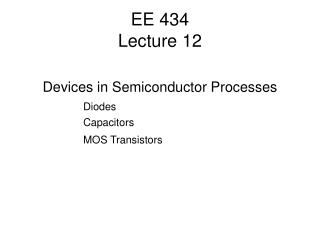Download PresentationEE 434 Lecture 12# EE 434 Lecture 12 - PowerPoint PPT Presentation

Download Presentation##### EE 434 Lecture 12

Download Policy: Content on the Website is provided to you AS IS for your information and personal use and may not be sold / licensed / shared on other websites without getting consent from its author. While downloading, if for some reason you are not able to download a presentation, the publisher may have deleted the file from their server.

- - - - - - - - - - - - - - - - - - - - - - - - - - - E N D - - - - - - - - - - - - - - - - - - - - - - - - - - -
##### Presentation Transcript

1. EE 434Lecture 12 Devices in Semiconductor Processes Diodes Capacitors MOS Transistors

2. Quiz 10 A “10K” resistor has a temperature coefficient of +80ppm/oC If the resistor was measured to be 9.83K at 20oC, what would be the resistor value at 80oC?

3. And the number is ….

4. Quiz 10 A “10K” resistor has a temperature coefficient of +80ppm/oC If the resistor was measured to be 9.83K at 20oC, what would be the resistor value at 80oC? Solution

5. Review from Last Time • Process Flow is a “recipe” for the process • Shows what can and can not be made • Gives insight into performance capabilities and limitations • Back-End Processes • Die attach options (eutectic, preform,conductive epoxy) • Stresses the die • Bonding • Wire bonding • Bump bonding • Packaging • Many packaging options • Package Costs can be large so defective die should be eliminated before packaging

6. Basic Devices and Device Models • Resistor • Diode • Capacitor • MOSFET • BJT

7. Diodes (pn junctions) N P Depletion region created that is ionized but void of carriers

8. pn Junctions N P Physical Boundary Separating n-type and p-type regions If doping levels identical, depletion region extends equally into n-type and p-type regions

9. pn Junctions N+ P- Physical Boundary Separating n-type and p-type regions Extends farther into p-type region if p-doping lower than n-doping

10. pn Junctions N- P+ Physical Boundary Separating n-type and p-type regions Extends farther into n-type region if n-doping lower than p-doping

11. pn Junctions N P I V

12. pn Junctions I N P V I V Diode Equation: JS= Sat Current Density A= Junction Cross Section Area VT=kT/q n is approximately 1

13. Basic Devices and Device Models • Resistor • Diode • Capacitor • MOSFET • BJT

14. Capacitors • Types • Parallel Plate • Fringe • Junction

15. Parallel Plate Capacitors A2 C A1 cond1 cond2 d insulator A = area of intersection of A1 & A2 One (top) plate intentionally sized smaller to determine C : Dielectric constant

16. Fringe Capacitors C d A is the area where the two plates are parallel Only a single layer is needed to make fringe capacitors

17. Capacitance Junction Capacitor C VD p d d n depletion region • Note: d is voltage dependent • capacitance is voltage dependent • usually parasitic caps • varicaps or varactor diodes exploit • voltage dep. of C Cj0: junction capacitance at VD = 0V B: barrier or built-in potential

18. Basic Devices and Device Models • Resistor • Diode • Capacitor • MOSFET • BJT

19. n-Channel MOSFET Poly Gate oxide n-active p-sub

20. n-Channel MOSFET Gate Drain Source L W LEFF Bulk

21. n-Channel MOSFET Poly Gate oxide n-active p-sub depletion region (electrically induced)

22. n-Channel MOSFET Operation and Model VDS ID VGS IG VBS IB Apply small VGS (VDS and VBS assumed to be small) ID=0 IG=0 IB=0 Depletion region electrically induced in channel Termed “cutoff” region of operation

23. n-Channel MOSFET Operation and Model VDS ID VGS IG VBS IB Increase VGS (VDS and VBS assumed to be small) ID=0 IG=0 IB=0 Depletion region in channel becomes larger

24. n-Channel MOSFET Operation and Model VDS Critical value of VGS that creates inversion layer termed threshold voltage, VT) ID VGS IG VBS IB (VDS and VBS small) Increase VGS more IDRCH=VDS IG=0 IB=0 Inversion layer forms in channel Inversion layer will support current flow from D to S Channel behaves as thin-film resistor

25. n-Channel MOSFET Operation and Model VDS ID VGS IG VBS IB (VDS and VBS small) Increase VGS more IDRCH=VDS IG=0 IB=0 Inversion layer in channel thickens RCH will decrease Termed “ohmic” or “triode” region of operation

26. Triode Region of Operation For VDS small

27. n-Channel MOSFET Operation and Model VDS ID VGS IG VBS IB (VBS small) Increase VDS ID=? IG=0 IB=0 Inversion layer thins near drain ID no longer linearly dependent upon VDS Still termed “ohmic” or “triode” region of operation

28. Triode Region of Operation For VDS larger

29. n-Channel MOSFET Operation and Model VDS ID VGS IG VBS IB (VBS small) Increase VDS even more ID=? IG=0 IB=0 Inversion layer disappears near drain Termed “saturation”region of operation Saturation first occurs when VDS=VGS-VT

30. Saturation Region of Operation For VDS at saturation

31. n-Channel MOSFET Operation and Model VDS ID VGS IG VBS IB (VBS small) Increase VDS even more (beyond VGS-VT) ID=? IG=0 IB=0 Nothing much changes !! Termed “saturation”region of operation

32. Saturation Region of Operation For VDS in Saturation

33. Model Summary Note: This is the third model we have introduced for the MOSFET